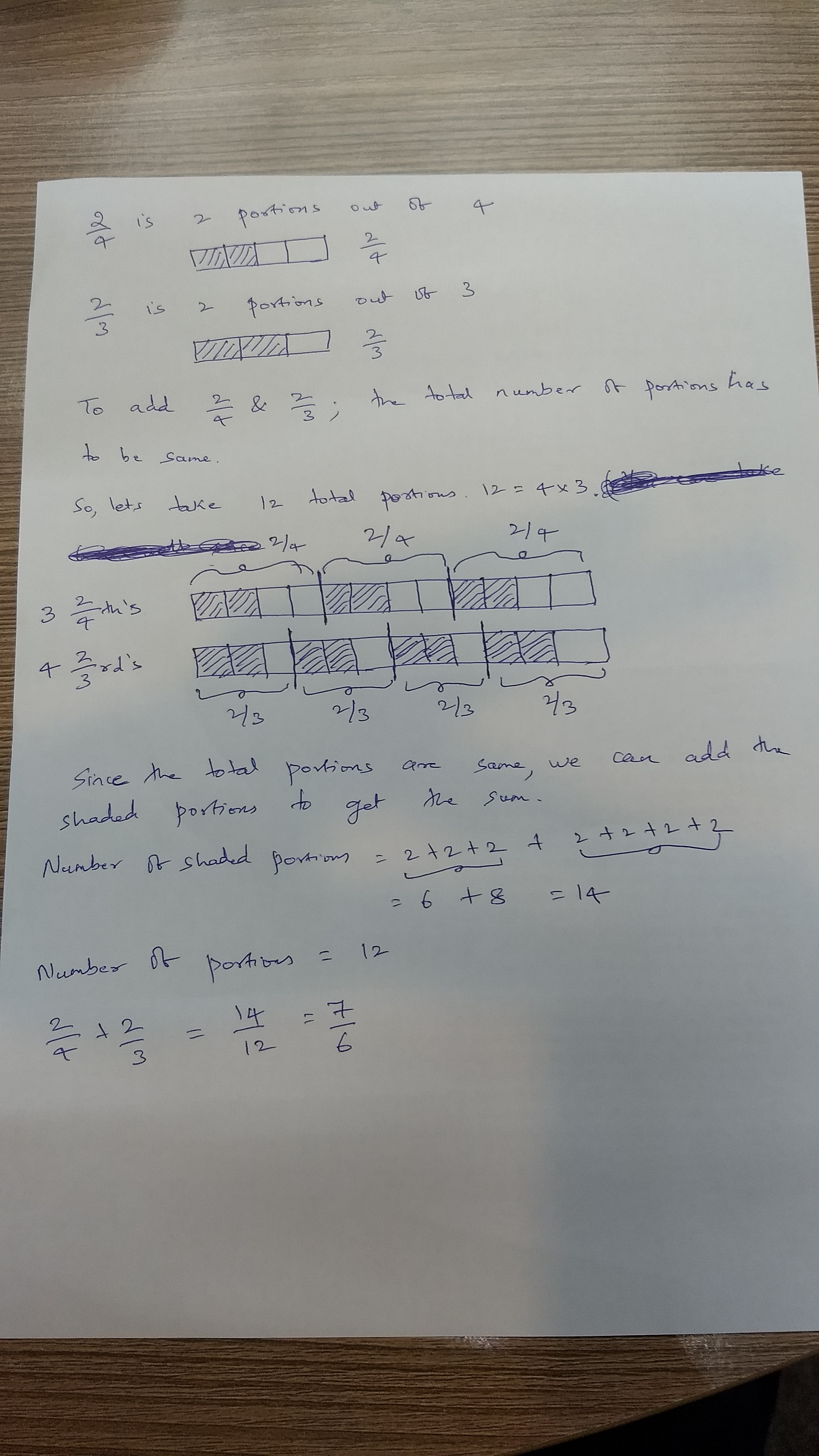Mahesh Godavarti
1
The steps to evaluate 2/4 + 2/3 are: 1. Rewrite each fraction in its simplest form, i.e. 1/2 + 2/3 2. Rewrite each fraction in a form that has the same denominator (the denominator should be least common multiple of the two denominators), i.e. 3/6 + 4/6 3. Simply add now that both fractions share the same denominator, i.e. 7/6 ------------------------ More explanation below: ------------------------ Adding fractions is easy, once we know exactly what they mean. Do we know how to evaluate 3/6 + 4/6? If yes, then we know how to evaluate 2/4 + 2/3. Because 2/4 is the same as 3/6 and 2/3 is the same as 4/6. Why is it easy to evaluate 3/6 + 4/6? First, 3/6 is the same as 3 one-sixths, and 4/6 is the same as 4 one-sixths. If there are 3 one-sixths and 4 one-sixths, they together make 7 one-sixths. Therefore, 3/6 + 4/6 = 7/6. It is easy to count how many portions there are if they are all the same of kind. So, what we need to do is to make sure we are counting the same kind of portions. 2/4 + 2/3 can be interpreted as 2 one-fourths and 2 one-thirds. This cannot simply be collapsed into a single "so many portions". So, the trick is to convert both fractions into describing the same portions. 2/4 which is 2 one-fourths is the same as 1 one-half, 3 one-sixths, 4 one-eights, or 5 one-tenths etc. 2/3 which is 2 one-thirds is the same as 4 one-sixths, 6 one-ninths, or 8 one-twelfths etc. Out of all these descriptions, the descriptions that describe the same portions are 3 one-sixths and 4 one-sixths. Therefore, 2/4 + 2/3 = 3/6 + 4/6 = 7/6.TheGOAT
0
thats to much for me I don't got time for thatMahesh Godavarti
0
Rewrote the answer for you. The first part is sufficient if you don't have time.Vivekanand Vellanki
0Visual representation of adding the fractions. These are useful to understand addition of fractions. It is better to use the short-cuts as outlined in Mahesh's response for a faster calculation.Sherlyn Casco Noperi
0
You cross multiply and get the answer.